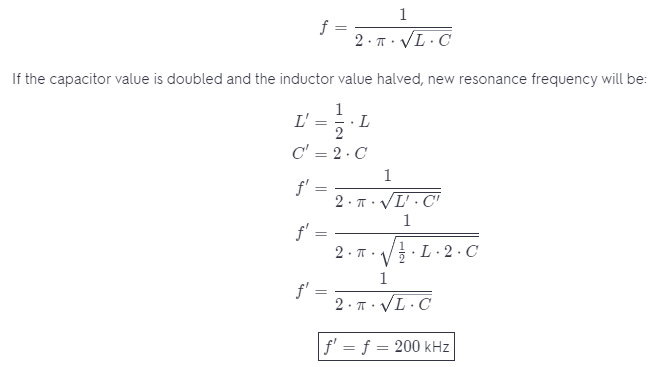# A Series RLC Circuit Has A 200 kHz Resonance Frequency.

We thoroughly check each answer to a question to provide you with the most correct answers. Found a mistake? Let us know about it through the REPORT button at the bottom of the page.

A series RLC circuit has a 200 kHz resonance frequency. What is the resonance frequency if the capacitor value is doubled and the inductor value is halved?

Answer: The resonance frequency will be the same.

## Explanation

The resonance frequency in a series RLC circuit is given by the equation: